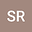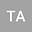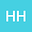Dynamic stall prediction of an airfoil using upwind-based numerical solution of non-viscous equations
•••• +1
• Seyed Esmaeil Razavi,
• Hussein Hassanpour,
• saeid soltani
Seyed Esmaeil Razavi
University of Tabriz

Corresponding Author:razavai@tabrizu.ac.ir

Author ProfileUniversity of Bonab
Author ProfileHussein Hassanpour
Iran University of Science and Technology
Author Profilesaeid soltani
Tabriz University
Author Profile## Abstract

Determination of the critical attack angle at which dynamic stall occurs has been a focus of research over the last few decades. This angle is usually determined by solving the Navier-Stokes equations, which includes viscosity, pressure, gravity, and acceleration terms. However, this article predicts the critical attack angle using Euler equations that do not contain viscous terms. The key contribution is the use of the Euler equations to capture the vortices and predict stall dynamics. The Euler equations are integrated and the resulting equations are discretized by the finite volume method. A first-order upwind-based method is used to calculate the convective fluxes at the cell boundaries in the finite volume approach. A NACA 0012 airfoil is chosen for this study at various attack angles with a Mach number of 0.3. Based on the justification of Crocco's theorem, the Euler equations successfully act as Navier-Stokes equations. The vortex patterns are found to behave independently of the artificial dissipation. All the vortices are successfully predicted using the inviscid governing equations. The obtained numerical results are validated by the existing experimental and numerical data.